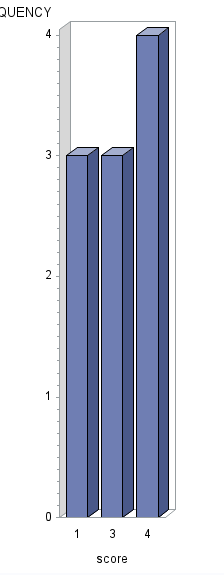## need 3dbar graph when knows x and y axis values

Hi , I have a data set and need the 3dbar graph . My SAS does not support proc sgplot.

data have;
infile datalines dlm = ",";
input student score;
datalines;
1,1
2,1
3,1
4,3
5,3
6,3
7,4
8,4
9,4
10,4
;
run;
%let max = 5;
/* Create a dummy set of all the desired groups */
data numbers;
do score = 1 to &max;
output;
end;
run;

/* Get the total count and each group's count */
proc summary data = have;
class score;
output out = counts;
run;
/* Combine and create the summary stats */
data want;
merge
counts (rename = (_FREQ_ = freq))
numbers;
by score;
/* Keep the total and cumulative total when new rows are loaded */
retain total cumsum;
/* Set up the total and delete the total row */
if _TYPE_ = 0 then do;
total = freq;
delete;
end;
/* Prevent missing values by adding 0 */
freq + 0;
/* Calculate stats */
pct = freq / total;
cumsum + freq;
cumpct = cumsum / total;
drop _TYPE_ total;
run;

data want(rename=(freq=n));
set want;
run;
proc gchart data=want;
vbar3d score/discrete raxis=n;
run;

Thanks

10 REPLIES 10

## Re: need 3dbar graph when knows x and y axis values

@daisy6 wrote:

Hi , I have a data set and need the 3dbar graph . My SAS does not support proc sgplot.

What version of SAS are you using then? That also limits the features for the Graphing Procedures?

## Re: need 3dbar graph when knows x and y axis values

It is SAS 9.3(32). When I run proc sgplot, the log window shows :

proc sgplot data=want;
208 vbar rawscore/ response=tn;
209 xaxis display=(nolabel);
210 yaxis grid;
211 run;

NOTE: Writing HTML Body file: sashtml.htm
NOTE: Since no format is assigned, the numeric category variable will use the default of BEST6.
NOTE: PROCEDURE SGPLOT used (Total process time):
real time 1.39 seconds
cpu time 0.26 seconds

ERROR: The Java proxy is not responding.
ERROR: The Java proxy's JNI call to start the VM failed.
ERROR: Unable to load the Java Virtual Machine. Please see the installation instructions or
ERROR: Unable to load the Java Virtual Machine. Please see the installation instructions or

Thanks

## Re: need 3dbar graph when knows x and y axis values

Basically, I need the bar graph even if the frequency=0. If do not consider frequency=0 value,

proc gchart data=have;
vbar3d score/discrete;
run;

works and I get capture picture which not included score=2 and 5.

But I need the bar chart with all the scores "1,2,3,4,5"

Can anyone help me out? Thanks a lot!Jay54
Meteorite | Level 14

## Re: need 3dbar graph when knows x and y axis values

SGPLOT is included with SAS 9.3 Base.  You are getting Java VM errors.  Run "proc javainfo all; run;" and post the log.

## Re: need 3dbar graph when knows x and y axis values

Here is the results:

NOTE: PROCEDURE JAVAINFO used (Total process time):
real time 0.23 seconds
cpu time 0.01 seconds

ERROR: The Java proxy is not responding.
ERROR: The Java proxy's JNI call to start the VM failed.
ERROR: Proc javainfo did not run correctly.
311 proc javainfo all; run;Jay54
Meteorite | Level 14

## Re: need 3dbar graph when knows x and y axis values

Clearly, your SAS software is not installed correctly.  You should contact your IT professionals, or SAS Technical Support.

## Re: need 3dbar graph when knows x and y axis values

Already reinstalled my SAS software and can use proc sgplot now. But the graph is not 3d bar shape if I use the code:

data have;
infile datalines dlm = ",";
input student score;
datalines;
1,1
2,1
3,1
4,3
5,3
6,3
7,4
8,4
9,4
10,4
;
run;
%let max = 5;
/* Create a dummy set of all the desired groups */
data numbers;
do score = 1 to &max;
output;
end;
run;

/* Get the total count and each group's count */
proc summary data = have;
class score;
output out = counts;
run;
/* Combine and create the summary stats */
data want;
merge
counts (rename = (_FREQ_ = freq))
numbers;
by score;
/* Keep the total and cumulative total when new rows are loaded */
retain total cumsum;
/* Set up the total and delete the total row */
if _TYPE_ = 0 then do;
total = freq;
delete;
end;
/* Prevent missing values by adding 0 */
freq + 0;
/* Calculate stats */
pct = freq / total;
cumsum + freq;
cumpct = cumsum / total;
drop _TYPE_ total;
run;

proc sgplot data=want;
vbar score/ response=freq;
xaxis display=(nolabel);
yaxis grid;
run;

proc sgplot data=want;
vbarparm category=score response=freq;
run;

Thank Sanjay!DanH_sas
SAS Super FREQ

## Re: need 3dbar graph when knows x and y axis values

Try using the DATASKIN option on the VBAR statement to give you a 3D-ish appearance, but maintain 2D readability. Try using values like PRESSED, GLOSS, or MATTE.

Hope this helps!

DanJay54
Meteorite | Level 14

## Re: need 3dbar graph when knows x and y axis values

SGPLOT does not support 3D bar shapes.  This is considered undesirable by many domain experts and thought leaders such as Tufte for analytical graphs.  However, we do recognize the need for some visual treatment and support the option DATASKIN.  You can try DATASKIN=PRESSED for 3D "looking" bars.  Or, other values such as GLOSS, SHEEN and MATTE.

http://blogs.sas.com/content/graphicallyspeaking/2016/12/19/axis-values-display/

## Re: need 3dbar graph when knows x and y axis values

Thank all of you! I will try it
Discussion stats
• 10 replies
• 892 views
• 0 likes
• 4 in conversation